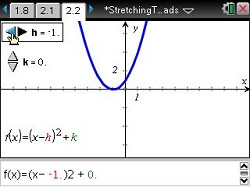# Activities

•##### Download

•• ##### Subject Area

• Math: Algebra II: Polynomials
• Math: College Algebra: Polynomial Functions

• ##### Author9-12

60 Minutes

• ##### Device
•TI-Nspire™ CX/CX II
•TI-Nspire™ CX CAS/CX II CAS
• TI-Nspire™
• TI-Nspire™ CAS
• ##### Software

TI-Nspire™
TI-Nspire™ CAS

3.0

## Stretching the Quads#### Activity Overview

In this activity, students will stretch and translate the parabola given by y = x2 and determine the effects on the equation. Students will also explore finding the vertex and zeros of a parabola and relate them to the equation.

#### Key Steps

•In this problem, students are told y = x2 is the basic equation for the standard form a parabola. Students then stretch the graph and observe how the equation changes. Students will make a connection between the curvature of the parabola and the equation. Several questions follow to determine if students have made a connection.